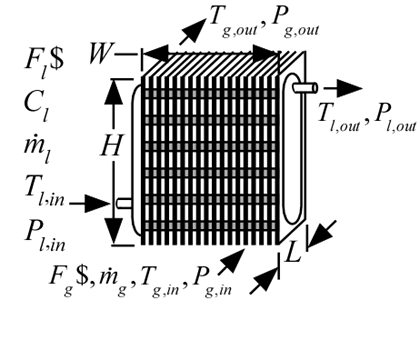Contents - index

HeatExchanger3_CLHeatExchanger3_CL models a circular finned tube heat exchanger in which a single phase liquid or brine (on the tube side) interacts with a single phase gas (on the fin side).  The procedure works with any type of fluid on the liquid side (including brines) and any type of fluid on the gas side except brines.  The procedure uses the compact heat exchanger library to determine the gas-side heat transfer and pressure drop characteristics and correlations for flow in a round tube to estimate these characteristics on the liquid side.  Pressure drop on both sides is calculated including both friction and head loss related to expansions/contractions.

Properties are assumed to be constant and are computed at the inlet temperatures.  Effectiveness is calculated assuming that heat exchanger behaves as an unmixed-unmixed cross-flow heat exchanger.

Inputs:

F_l\$:  Liquid string identifier

C_l:  concentration of liquid (%) (only used for a brine - otherwise set to 0)

m_dot_l:  total mass flow rate of liquid (kg/s or lbm/hr); this value is divided by the number of tubes and multiplied by the number of tube passes to get the mass flow rate per tube

T_l_in:  inlet temperature of liquid (K, C, R, or F)

P_l_in:  inlet pressure of liquid (bar, atm, Pa, kPa, or MPa)

F_g\$:  Gas string identifier

m_dot_g:  total mass flow rate of gas (kg/s or lbm/hr)

T_g_in:  inlet temperature of gas (K, C, R, or F)

P_g_in:  inlet pressure of gas (bar, atm, Pa, kPa, or MPa)

W:  width of heat exchanger face parallel to tubes (m or ft)

H:  height of heat exchanger face perpendicular to tubes (m or ft)

L:  length of heat exchanger in flow direction (m or ft)

HXm\$:  string identifier for compact heat exchanger geometry

HXg\$:  string identifier for material used for fins;

if set to 'NA' then fins will be assumed to be 100% efficient

th_tb:  tube thickness (m or ft); if set to a negative number then a reasonable value is assumed

RR:  relative roughness of inner surface of tube

N_pass:  number of tube passes (-)

Outputs:

T_l_out:  liquid outlet temperature (K, C, R, or F)

P_l_out:  liquid outlet pressure (bar, atm, Pa, kPa, or MPa)

T_g_out:  gas outlet temperature (K, C, R, or F)

P_g_out:  gas outlet pressure (bar, atm, Pa, kPa, or MPa)

Q_dot:  heat transfer rate (W, kW, or Btu/hr)

note that positive indicates from liquid to gas, negative indicates from gas to liquid

eff:  effectiveness

NTU:  Number of transfer units

eta_f: f in efficiency"

Example:

\$UnitSystem SI Mass J K Pa

F_l\$='Engine_Oil_unused'

C_l=0 [%]

m_dot_l=0.015 [kg/s]

T_l_in=350 [K]

P_l_in=110000 [Pa]

F_g\$='Air'

m_dot_g=0.5 [kg/s]

T_g_in=300 [K]

P_g_in=110000 [Pa]

W=0.4 [m]

H=0.3[m]

L=0.1 [m]

HXg\$='fc_tubes_sCF-88-10Ja'

HXm\$='Copper'

N_pass=1

th_tb=0.035 [inch]*convert(inch,m)

RR=0 [-]

Call heatexchanger3_cl(F_l\$, C_l, m_dot_l, T_l_in, P_l_in, F_g\$, m_dot_g, T_g_in, P_g_in, W, H, &

L, HXg\$, HXm\$, th_tb, RR, N_pass: T_l_out, P_l_out, T_g_out, P_g_out, Q_dot, eff, NTU, eta_f)

{Solution:

T_l_out = 328.7 [K]

P_l_out = 109997 [Pa]

T_g_out = 301.3 [K]

P_g_out = 109949 [Pa]

Q_dot = 675.8 [W]

eff = 0.4245 [-]

NTU = 0.5654

eta_f = 0.9520}